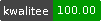++ed by:
1 non-PAUSE user
and 2 contributors

# NAME

Set::Similarity - similarity measures for sets# SYNOPSIS

`````` use Set::Similarity::Dice;

# object method
my \$dice = Set::Similarity::Dice->new;
my \$similarity = \$dice->similarity('Photographer','Fotograf');

# class method
my \$dice = 'Set::Similarity::Dice';
my \$similarity = \$dice->similarity('Photographer','Fotograf');

# from 2-grams
my \$width = 2;
my \$similarity = \$dice->similarity('Photographer','Fotograf',\$width);

# from arrayref of tokens
my \$similarity = \$dice->similarity(['a','b'],['b']);

# from hashref of features
my \$bird = {
wings    => true,
eyes     => true,
feathers => true,
hairs    => false,
legs     => true,
arms     => false,
};
my \$mammal = {
wings    => false,
eyes     => true,
feathers => false,
hairs    => true,
legs     => true,
arms     => true,
};
my \$similarity = \$dice->similarity(\$bird,\$mammal);

# from arrayref sets
my \$bird = [qw(
wings
eyes
feathers
legs
)];
my \$mammal = [qw(
eyes
hairs
legs
arms
)];
my \$similarity = \$dice->from_sets(\$bird,\$mammal);``````

# DESCRIPTION

This is the base class including mainly helper and convenience methods.

## Overlap coefficient

( A intersect B ) / min(A,B)

## Jaccard Index

The Jaccard coefficient measures similarity between sample sets, and is defined as the size of the intersection divided by the size of the union of the sample sets

( A intersect B ) / (A union B)

The Tanimoto coefficient is the ratio of the number of features common to both sets to the total number of features, i.e.

( A intersect B ) / ( A + B - ( A intersect B ) ) # the same as Jaccard

The range is 0 to 1 inclusive.

## Dice coefficient

The Dice coefficient is the number of features in common to both sets relative to the average size of the total number of features present, i.e.

( A intersect B ) / 0.5 ( A + B ) # the same as sorensen

The weighting factor comes from the 0.5 in the denominator. The range is 0 to 1.

# METHODS

All methods can be used as class or object methods.

## new

``  \$object = Set::Similarity->new();``

## similarity

``  my \$similarity = \$object->similarity(\$any1,\$any1,\$width);``

`\$any` can be an arrayref, a hashref or a string. Strings are tokenized into n-grams of width `\$width`.

`\$width` must be integer, or defaults to 1.

## from_tokens

``  my \$similarity = \$object->from_tokens(['a','b'],['b']);``

## from_sets

``  my \$similarity = \$object->from_sets(['a'],['b']);``

Croaks if called directly. This method should be implemented in a child module.

## intersection

``  my \$intersection_size = \$object->intersection(['a'],['b']);``

## uniq

``  my @uniq = \$object->uniq(['a','b']);``

Transforms an arrayref of strings into an array of unique elements.

## combined_length

``  my \$set_size_sum = \$object->combined_length(['a'],['b']);``

## min

``  my \$min_set_size = \$object->min(['a'],['b']);``

## ngrams

``````  my @monograms = \$object->ngrams('abc');
my @bigrams = \$object->ngrams('abc',2);``````

## _any

``  my \$arrayref = \$object->_any(\$any,\$width);``

Set::Similarity::Cosine

Set::Similarity::Dice

Set::Similarity::Jaccard

Set::Similarity::Overlap

Bag::Similarity doing the same for bags or multisets.

Text::Levenshtein for distance measures of strings, and a very overview of similar modules,

http://en.wikipedia.org/wiki/String_metric for an overview of similarity measures.

Cluster::Similarity for clusters.

# SOURCE REPOSITORY

http://github.com/wollmers/Set-Similarity

# AUTHOR

Helmut Wollmersdorfer, <helmut@wollmersdorfer.at>